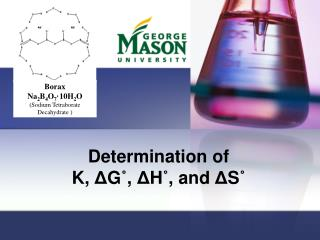DownloadDownload PresentationDetermination of K, Δ G ˚ , Δ H ˚ , and Δ S ˚

Determination of K, Δ G ˚ , Δ H ˚ , and Δ S ˚

Download PresentationDetermination of K, Δ G ˚ , Δ H ˚ , and Δ S ˚

- - - - - - - - - - - - - - - - - - - - - - - - - - - E N D - - - - - - - - - - - - - - - - - - - - - - - - - - -
Presentation Transcript

1. Borax Na2B4O7·10H2O (Sodium Tetraborate Decahydrate ) Determination of K, ΔG˚, ΔH˚, and ΔS˚

2. Quiz Question # 4 • Look at following equation to write down your Ksp:

3. First things first… • Safety: • Put bags away • Goggles • Lab Jacket • Turn in Lab Reports • LAB!

4. Borax Equilibrium

5. Let’s Simplify!!

6. Today’s Rxn

7. Gibbs Free Energy (ΔG), Enthalpy (ΔH), and Entropy (ΔS) y = m x + b

8. Changes in Procedure • Each group does one temp (assigned). 2 trials. • Add 2 scoops of borax and 75 mL of distilled water to a beaker • Heat the solution (<50 °C) so that the solution is saturated. Heat slowly! No need to speed up the heating process. • Once slightly above assigned temperature, take off hotplate. • If all the solid has dissolved, add some more borax to beaker. • Pour 5 mL of the solution into a graduated cylinder once the solution is at the desired temperature. Record volume to tenths place. • Be sure to record temperature to the tenths place.

9. Procedure (continued) • Add the 5 mL of solution to an Erlenmeyer with 50 mL distilled water, 5 drops bromocresol green, mix thoroughly, add a little distilled water to rinse the graduated cylinder into the Erlenmeyer (Quantitative Transfer) • Titrate with HCl (clean/rinse buret and fill w/ ~20 mL HCl) • REPEAT! The above steps for two more titrations. • DO NOT try to prepare three samples at once, unless you can guarantee the temperature of the borax solution will not change dramatically!

10. Turn in class: • Pre-lab, filled in data tables, observations • Type in you and your partner’s data in class computers on opened excel spreadsheet

11. Formal Lab Report – due in two weeks • Table 1, 2 – for each trial solve for: • Table 4 – average the trials to find (for each temp):

12. Data Tables (con’t) Table 5 – use ΔH and ΔS to solve for ΔG for each Temp

13. Graph: Make using Table 4 data

14. Calculations • Find molarity of borax (mol/L) [Borax] • Find moles borate (use moles HCl) • Find volume of solution (borate + HCl) • Find Ksp • Ksp = 4[Borax]

15. Calculations • Determine dH (kJ/mol) and dS (J/mol K) from this graph. • note: dH = ΔH • Use trendline from graph y = mx + b

16. Calculations con’t • Calculate the values for dG for each sample using: • dG = -RT ln Ksp • used for table 4 • dG = dH - TdS with your solved values for dH and dS • used for table 5

17. Discussion Analysis • Consider the following: • Compare solving delta G between the two equations, which yielded better results? • Consider the precision of the graphs